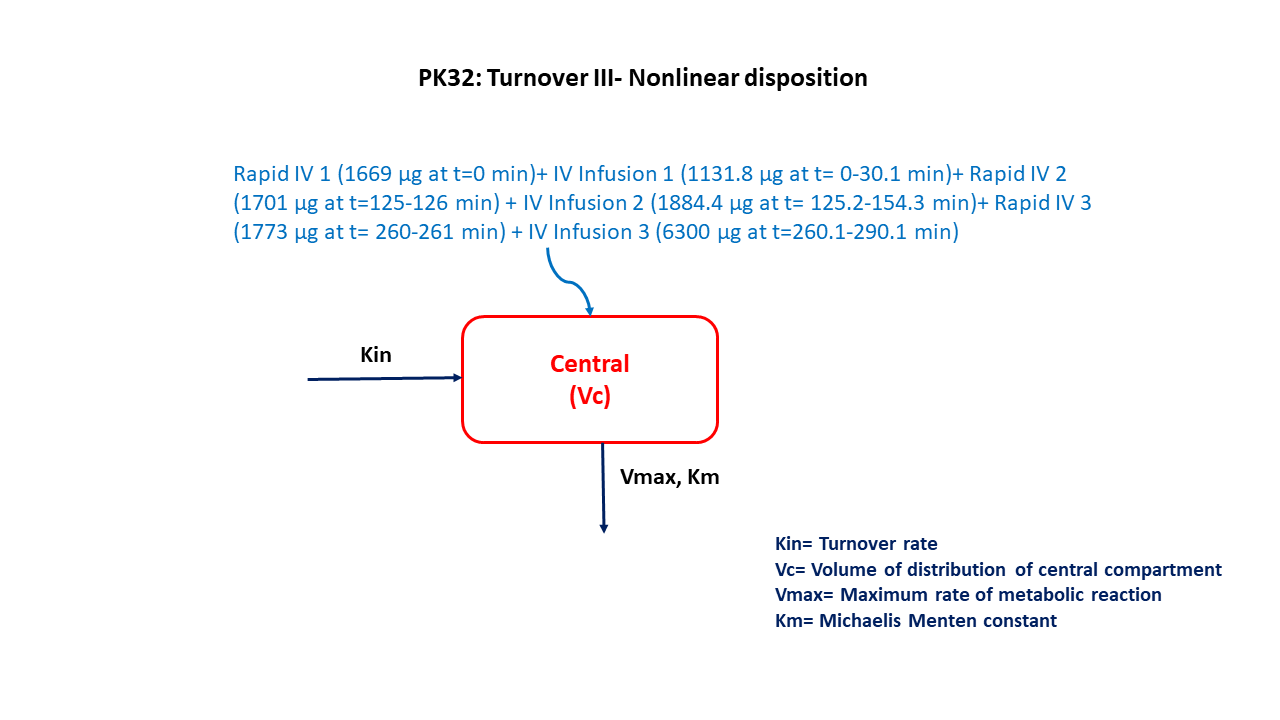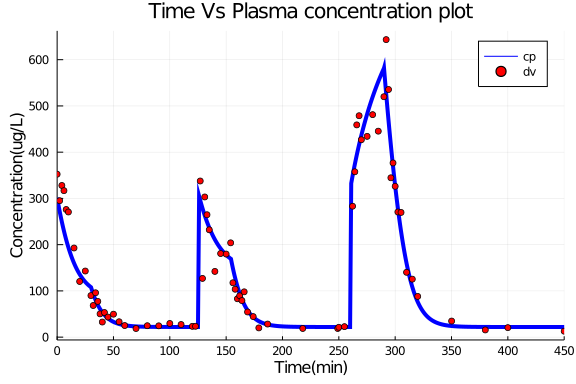# Exercise PK32 - Turnover III Nonlinear disposition

### Background

• Structural model - One compartment with zero order input and non linear elimination

• Route of administration - IV infusion

• Dosage Regimen - Multiple intravenous infusions (Three sets of Rapid iv infusion followed by slow iv infusion)

• Number of Subjects - 1### Learning Outcomes

We will learn to analyze data obtained after Multiple infusions of an endogenous compound with non-linear disposition

### Objectives

• To bulid a one compartment model for an endogenous compound with non-linear disposition

• To use final parameter estimates and design a multiple infusion dosage regimen

• To simulate and plot a single subject with predefined time points.

### Libraries

Call the "necessary" libraries to get started.

using Pumas
using Plots
using CSV
using StatsPlots
using Random


### Model

One compartment model for an endogenous compound with non-linear disposition

pk_32        = @model begin
@param begin
tvvc     ∈ RealDomain(lower=0)
tvvmax   ∈ RealDomain(lower=0)
tvkm     ∈ RealDomain(lower=0)
tvkin    ∈ RealDomain(lower=0)
Ω        ∈ PDiagDomain(4)
σ        ∈ RealDomain(lower=0)
end

@random begin
η        ~ MvNormal(Ω)
end

@pre begin
Vc       = tvvc*exp(η)
Vmax     = tvvmax*exp(η)
Km       = tvkm*exp(η)
Kin      = tvkin*exp(η)
#CL      = Vmax/(Km+(Central/Vc))
end

@init begin
Central  = Kin/((Vmax/Km)/Vc)
end

@dynamics begin
Central' = Kin - (Vmax/(Km+Central/Vc))*(Central/Vc)
end

@derived begin
cp       = @. Central/Vc
dv       ~ @. Normal(cp, sqrt(cp^2*σ))
end
end

PumasModel
Parameters: tvvc, tvvmax, tvkm, tvkin, Ω, σ
Random effects: η
Covariates:
Dynamical variables: Central
Derived: cp, dv
Observed: cp, dv


### Parameters

The parameters are as given below. tv represents the typical value for parameters.

• Vc - Volume of Central Compartment (L)

• Vmax- Maximum metabolic capacity (μg/min)

• Km - Michaelis- menten constant (μg/L)

• Kin - Rate of synthesis,Turnover rate (μg/min)

• Ω - Between Subject Variability

• σ - Residual error

param = (tvvc   = 5.94952,
tvvmax = 361.502,
tvkm   = 507.873 ,
tvkin  = 14.9684,
Ω      = Diagonal([0.00,0.00,0.00,0.00]),
σ      = 0.05)

(tvvc = 5.94952, tvvmax = 361.502, tvkm = 507.873, tvkin = 14.9684, Ω = [0.
0 0.0 0.0 0.0; 0.0 0.0 0.0 0.0; 0.0 0.0 0.0 0.0; 0.0 0.0 0.0 0.0], σ = 0.05
)


### Dosage Regimen

DosageRegimen (DR) = Three sets of rapid intravenous infusion followed by slow intravenous infusion as followed

• IV bolus of 1669 μg (Time=0 min) followed by IV infusion of 1131.8 μg (Time= 0-30.1 min)

• IV infusion of 1701 μg (Time= 0-30.1 min) followed by IV infusion of 1884.4 μg (Time= 125.2-154.3 min)

• IV infusion of 1773 μg (Time= 260-261 min) followed by IV infusion of 6300 μg (Time= 260.1-290.1 min)

IVinfRapid = DosageRegimen([1669,1701,1733], time=[0,125,260], cmt=[1,1,1], duration=[0,1,1])
IVinfSlow  = DosageRegimen([1131.8,1884.4,6300],time=[0,125.2,260.1], cmt=[1,1,1], duration=[30.1,29.1,30])
DR         = DosageRegimen([IVinfRapid,IVinfSlow])
sub1       = Subject(id=1, events=DR)

Subject
ID: 1
Events: 11


### Simulation

To simulate plasma concentration for single subject with the specific observation time points for a given dosage regimen 'DR'

sim  =  simobs(pk_32,sub1,param,obstimes=0:0.01:450)


### DataFrame & Plot

plasmadata    = DataFrame(sim)
dropmissing!(plasmadata, :cp)
plasmadata_dv = filter(x -> x.time in [0,2.23,4.2,6.05,8.03,10,15,20,25,30,32,34.1,36.1,38.1,40.1,42,45.1,50,
55,60,70,80,90.2,100,110,120,122.8,127,129,131,133,135,140,145.1,150,154,156,158,160,162,164,166,169,174,179,
186.8,218,249,250,255,262.2,264.2,265.9,268,270,275.1,280,285,290,292,294.1,296.2,298.1,300,302.4,305.2,310.1,
315.2,320,350.1,380,400,450], plasmadata)
@df plasmadata plot(:time, :cp, color= [:blue], linewidth=4,
label= "cp", ylabel="Concentration(ug/L)", xlabel="Time(min)",
title= "Time Vs Plasma concentration plot")
@df plasmadata_dv scatter!(:time, :dv,color= [:red], label= "dv",
xticks=[0,50,100,150,200,250,300,350,400,450], xlims=(0,450))